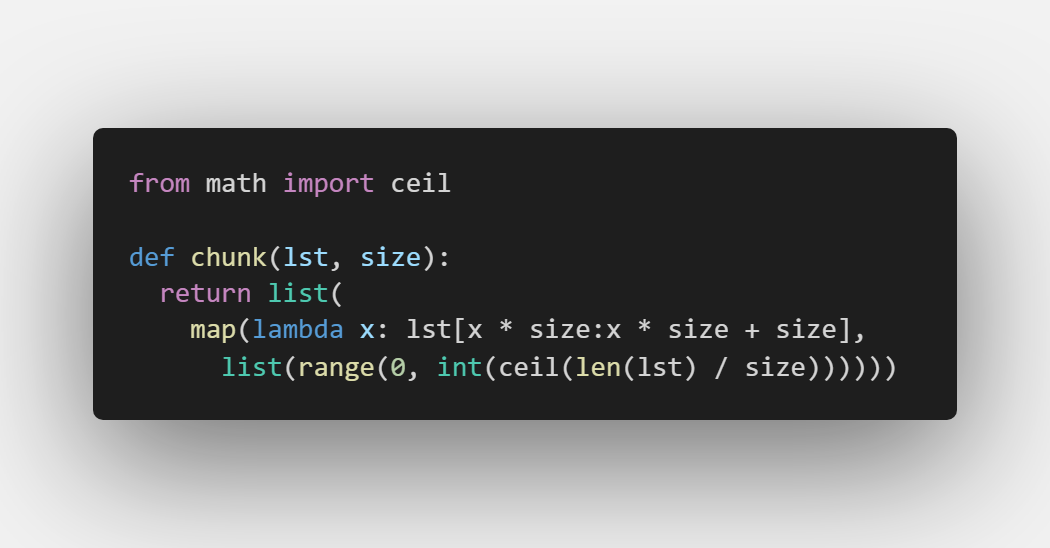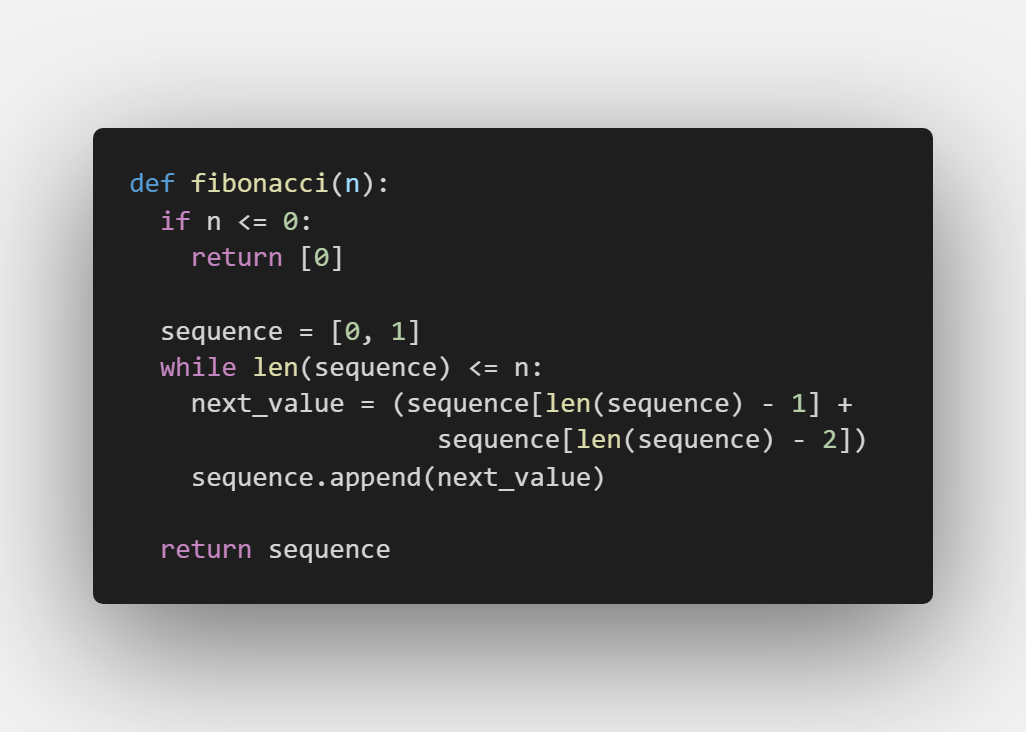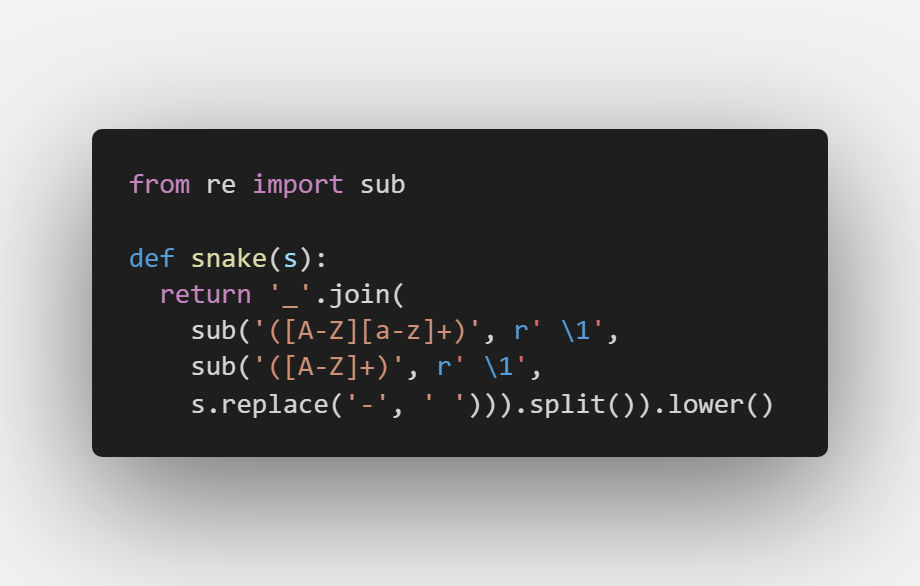### 今天给大家带来一些30秒就能学会的代码片段，这些代码潜力无限，蕴含了丰富的python编程思维，应用领域非常广泛，而且学起来非常简单。

1.”二维列表”

``def initialize_2d_list(w, h, val=None):    return [[val for x in range(w)] for y in range(h)]``

``>>> initialize_2d_list(2,2)[[None, None], [None, None]] >>> initialize_2d_list(2,2,0)[[0, 0], [0, 0]]``

2.函数切割数组

``def bifurcate_by(lst, fn):    return [      [x for x in lst if fn(x)],      [x for x in lst if not fn(x)]    ]``

``>>> bifurcate_by(['beep', 'boop', 'foo', 'bar'], lambda x: x == 'b') [['beep', 'boop', 'bar'], ['foo']]``

3.”交集点”

``def interp_by(a, b, fn):    _b = set(map(fn, b))    return [item for item in a if fn(item) in _b]``

``>>> from math import floor>>> interp_by([2.1, 1.2], [2.3, 3.4],floor)[2.1]``

4.最大值下标

``def max_element_index(arr):    return arr.index(max(arr))``

``>>> max_element_index([5, 8, 9, 7, 10, 3, 0])4``

5.数组对称差

``def symmetric_difference(a, b):    _a, _b = set(a), set(b)    return [item for item in a if item not in _b] + [item for item in b if item not in _a]``

``>>> symmetric_difference([1, 2, 3], [1, 2, 4])[3, 4]``

6.”夹数”

``def clamp_number(num,a,b):    return max(min(num, max(a,b)),min(a,b))``

``>> clamp_number(2,3,10)3 >> clamp_number(7,3,10)7 >> clamp_number(124,3,10)10``

7.键值映射

``def map_values(obj, fn):    ret = {}    for key in obj.keys():        ret[key] = fn(obj[key])    return ret``

``>>> users = {...   'fred': { 'user': 'fred', 'age': 40 },...   'pebbles': { 'user': 'pebbles', 'age': 1 }... } >>> map_values(users, lambda u : u['age']){'fred': 40, 'pebbles': 1} >>> map_values(users, lambda u : u['age']+1){'fred': 41, 'pebbles': 2}``

8.大小写转换

upper_rest参数：设定是否将除首字母外的其他字母大小写转换。

``def decapitalize(s, upper_rest=False):    return s[:1].lower() + (s[1:].upper() if upper_rest else s[1:])``

``>>> decapitalize('FooBar')'fooBar' >>> decapitalize('FooBar', True)'fOOBAR'``

9.同键求和

``def sum_by(lst, fn):    return sum(map(fn,lst))``

``>>> sum_by([{ 'n': 4 }, { 'n': 2 }, { 'n': 8 }], lambda v : v['n'])14``

10.一行代码求出现次数

``def count_occurrences(lst, val):    return len([x for x in lst if x == val and type(x) == type(val)])``

``>>> count_occurrences([1, 1, 2, 1, 2, 3], 1)3``

11.数组再分组``chunk([1,2,3,4,5],2)# [[1,2],[3,4],5]``

return中，map的第二个参数是一个列表，map会将列表中的每一个元素用于调用第一个参数的 function 函数，返回包含每次 function 函数返回值的新列表。

12.数字转数组

``def digitize(n):    return list(map(int, str(n)))``

``digitize(123)# [1, 2, 3]``

13.非递归斐波那契``fibonacci(7)# [0, 1, 1, 2, 3, 5, 8, 13]``

14.下划线化字符串``snake('camelCase')# 'camel_case' snake('some text')# 'some_text' snake('some-mixed_string With spaces_underscores-and-hyphens')# 'some_mixed_string_with_spaces_underscores_and_hyphens' snake('AllThe-small Things')# "all_the_small_things"``

re.sub用于替换字符串中的匹配项。这里其实是一个“套娃”用法，一开始可能不太好理解，需要慢慢理解。

Python实用宝典 (pythondict.com)